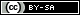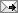## Gavin R. Putland,  BE PhD

 Friday, May 08, 2020 (Comment)

# Huygens' principle: Simple mechanistic reason for the phase advance of the secondary sourcesThis page uses MathJax™ to display equations and mathematical symbols. It requires JavaScript™ and an up-to-date browser.

Consider the “primary” wave field \begin{equation}\tag{1}\psi(r,t)=\frac{f(t-r/c)}{r}\,,\end{equation} where $$r$$ is distance from the source, $$t$$ is time, $$f(t)$$ is a function characterizing the source, and $$c$$ is constant. Let $$\psi_r$$ and $$\dot{\psi}$$ denote the partial derivatives of $$\psi$$ w.r.t. $$r$$ and $$t$$, respectively, and let $$dS$$ be an area element of the sphere of radius $$r$$ centered on the source. Evaluating and comparing the partial derivatives, we readily obtain \begin{equation}\tag{2}-\psi_r\;\!dS=\bigg(\frac{\dot\psi}{\,c\,}+\frac{\psi}{\,r\,}\bigg)\,dS\,.\end{equation} For the secondary source corresponding to $$dS$$, let the average (over all directions) of the wave function at distance $$s$$ be \begin{equation}\tag{3}\varphi(s,t)=\frac{g(t-s/c)}{s}\,,\end{equation} where $$g(t)$$ is a function to be determined, characterizing the secondary source. Let $$A$$ be the area of a sphere of radius $$s$$ centered on $$dS$$, so that \begin{equation}\tag{4}A=4\pi s^2.\end{equation} Differentiating (3) w.r.t. $$s$$ and multiplying by (4), we get \begin{equation}\tag{5}-\varphi_s\;\!A=4\pi\bigg(\frac{s\;\!g'(t{-}s/c)}{c}+g\big(t{-}s/c\big)\bigg)\end{equation} whence, as $$s\!\to\!0$$, \begin{equation}\tag{6}-\varphi_s\;\!A\to 4\pi g(t)\,.\end{equation} If  $$-\psi_r$$ and $$-\varphi_s$$ represent per-unit-area flows (e.g., if the wave function is a velocity potential) or time-derivatives thereof (e.g., for sound waves in which the wave function is pressure relative to equilibrium), then, by conservation of whatever is flowing, we can equate the left-hand sides of (2) and (6), hence their right-hand sides, obtaining \begin{equation}\tag{7}g(t)=\frac{1}{4\pi}\bigg(\frac{\dot\psi}{\,c\,}+\frac{\psi}{\,r\,}\bigg)\,dS\,.\end{equation} The term in $$\dot{\psi}$$, which is advanced in phase by 90° w.r.t. $$\psi$$ for sinusoidal $$f(t)$$, is dominant for sufficiently large $$r$$.

In the exact Kirchhoff diffraction formula , the factor corresponding to $$g(t)$$, but also accounting for directionality, is \begin{equation}\tag{8}\frac{1}{4\pi}\Bigg\{\frac{\cos(n,r)-\cos(n,s)}{c}\,\dot\psi\,+\,\bigg(\frac{\cos(n,r)}{r}-\frac{\cos(n,s)}{s}\bigg)\psi\,\Bigg\}\,dS\,,\end{equation} where $$n$$ is the coordinate normal to $$dS$$ (and $$s$$ is measured to $$dS$$). Here again the term in $$\dot\psi$$ is dominant for sufficiently large $$r$$ (and $$s$$).

Acknowledgment:  This post was inspired in part by reference , but takes a different approach.

#### ReferencesTweetReturn to Contents